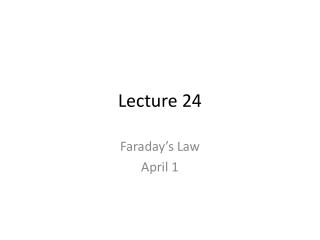# Lecture 24 - PowerPoint PPT PresentationDownload PresentationLecture 24

Lecture 24
Download Presentation## Lecture 24

- - - - - - - - - - - - - - - - - - - - - - - - - - - E N D - - - - - - - - - - - - - - - - - - - - - - - - - - -
##### Presentation Transcript

1. Lecture 24 Faraday’s Law April 1

2. Electromagnetic Induction Slide 25-8

3. Motional emf Slide 25-9

4. Checking Understanding A loop of wire of area A is tipped at an angle to a uniform magnetic field B. The maximum flux occurs for an angle . What angle will give a flux that is ½ of this maximum value? A. B. C. D. Slide 25-11

5. Lenz’s Law Lenz’s law There is an induced current in a closed, conducting loop if and only if the magnetic flux through the loop is changing. The direction of the induced current is such that the induced magnetic field opposes the change in the flux. Slide 25-13

6. Checking Understanding A long conductor carrying a current runs next to a loop of wire. The current in the wire varies as in the graph. Which segment of the graph corresponds to the largest induced current in the loop? Slide 25-16

7. Largest current in loop? • A • B • C • D • E • F

8. A magnetic field goes through a loop of wire, as below. If the magnitude of the magnetic field is increasing, what can we say about the current in the loop? • The loop has a clockwise current • The loop has a counter clockwise current • There is no current in the loop

9. A magnetic field goes through a loop of wire, as below. If the magnitude of the magnetic field is decreasing, what can we say about the current in the loop? • The loop has a clockwise current • The loop has a counter clockwise current • The loop has no current

10. Checking Understanding • A battery, a loop of wire, and a switch make a circuit below. A second loop of wire sits directly below the first. Just before the switch is closed, what can we say about the current in the lower loop? • A. The loop has a clockwise current. • B. The loop has a counterclockwise current. • C. The loop has no current. Slide 25-24

11. Checking Understanding • A battery, a loop of wire, and a switch make a circuit below. A second loop of wire sits directly below the first. Immediately after the switch is closed, what can we say about the current in the lower loop? • A. The loop has a clockwise current. • B. The loop has a counterclockwise current. • C. The loop has no current. Slide 25-26

12. Checking Understanding • A battery, a loop of wire, and a switch make a circuit below. A second loop of wire sits directly below the first. Long after the switch is closed, what can we say about the current in the lower loop? • A. The loop has a clockwise current. • B. The loop has a counterclockwise current. • C. The loop has no current. Slide 25-28

13. Checking Understanding • A battery, a loop of wire, and a switch make a circuit below. A second loop of wire sits directly below the first. Immediately after the switch is reopened, what can we say about the current in the lower loop? A. The loop has a clockwise current. • B. The loop has a counterclockwise current. • C. The loop has no current. Slide 25-30

14. Friday • Start electromagnetic waves • MONDAY: Review